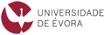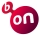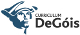Please use this identifier to cite or link to this item: `http://hdl.handle.net/10174/29356`

 Title: Symmetric decomposition of the associated graded algebra of an Artinian Gorenstein algebra Authors: Iarrobino, AnthonyMacias Marques, Pedro Keywords: Artinian GorensteinLocal algebraGorenstein sequenceSymmetric decompositionDeformationNormal form of dual generator Issue Date: 2021 Publisher: Journal of Pure and Applied Algebra Citation: Anthony Iarrobino, Pedro Macias Marques, Symmetric Decomposition of the Associated Graded Algebra of an Artinian Gorenstein Algebra, Journal of Pure and Applied Algebra 225 (2021), n.º 3, 106496, 49 pp. Abstract: We study the symmetric subquotient decomposition of the associated graded algebras A∗ of a non-homogeneous commutative Artinian Gorenstein (AG) algebra A. This decomposition arises from the stratification of A∗ by a sequence of ideals A∗ = CA(0), CA(1),... whose successive quotients Q(a) = C(a)/C(a+1) are reflexive A∗ modules. These were introduced by the first author [47,48], developed in the Memoir , and have been used more recently by several groups, especially those interested in short Gorenstein algebras, and in the scheme length (cactus rank) of forms. For us a Gorenstein sequence is an integer sequence H occurring as the Hilbert function H = H(A) for an AG algebra A, that is not necessarily homogeneous. Such a Hilbert function H(A) is the sum of symmetric non-negative sequences HA(a) = H QA(a) , each having center of symmetry (j − a)/2 where j is the socle degree of A: we call these the symmetry conditions, and the decomposition D(A) = HA(0), HA(1),... the symmetric decomposition of H(A) (Theorem 1.4). We here study which sequences may occur as the summands HA(a): in particular we construct in a systematic way examples of AG algebras A for which HA(a) can have interior zeroes, as HA (a) = (0, s, 0, . . . , 0, s, 0). We also study the symmetric decomposition sets D(A), and in particular determine which sequences HA(a) can be non-zero when the dual generator is linear in a subset of the variables (Theorem 4.1). Several groups have studied “exotic summands” of the Macaulay dual generator F : these are summands that involve more successive variables than would be expected from the symmetric decomposition of the Hilbert function H(A). Studying these, we recall a normal form for the Macaulay dual generator of an AG algebra that has no “exotic” summands (Theorem 2.7). We apply this to Gorenstein algebras that are connected sums (Section 2.4). We give throughout many examples and counterexamples, and conclude with some open questions about symmetric decomposition. URI: https://doi.org/10.1016/j.jpaa.2020.106496http://hdl.handle.net/10174/29356 Type: article Appears in Collections: MAT - Publicações - Artigos em Revistas Internacionais Com Arbitragem Científica

Files in This Item:

File Description SizeFormat
2021JPureApplAlgebraIarrobinoMaciasMarques.pdf992.42 kBAdobe PDFView/Open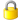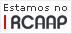DSpace Software, version 1.6.2 Copyright © 2002-2008 MIT and Hewlett-Packard - Feedback